Chemical Thermodynamics

# Chemical Thermodynamics

Test Description

## 20 Questions MCQ Test GATE Chemistry Mock Test Series | Chemical Thermodynamics

Chemical Thermodynamics for Chemistry 2022 is part of GATE Chemistry Mock Test Series preparation. The Chemical Thermodynamics questions and answers have been prepared according to the Chemistry exam syllabus.The Chemical Thermodynamics MCQs are made for Chemistry 2022 Exam. Find important definitions, questions, notes, meanings, examples, exercises, MCQs and online tests for Chemical Thermodynamics below.
Solutions of Chemical Thermodynamics questions in English are available as part of our GATE Chemistry Mock Test Series for Chemistry & Chemical Thermodynamics solutions in Hindi for GATE Chemistry Mock Test Series course. Download more important topics, notes, lectures and mock test series for Chemistry Exam by signing up for free. Attempt Chemical Thermodynamics | 20 questions in 60 minutes | Mock test for Chemistry preparation | Free important questions MCQ to study GATE Chemistry Mock Test Series for Chemistry Exam | Download free PDF with solutions
 1 Crore+ students have signed up on EduRev. Have you?
Chemical Thermodynamics - Question 1

### For which set of ΔH• and ΔS- will the reaction be spontaneous only at high temperature

Detailed Solution for Chemical Thermodynamics - Question 1

At high temperature, ΔG = ΔH - TΔS
Only, ΔH = +ve , ΔS = +ve, T = high
Reaction spontaneous, ΔG = -ve

*Answer can only contain numeric values
Chemical Thermodynamics - Question 2

### The final temperature of one mole of gas at 200 atm an d 19°C as it is forced through a porous plug to a final pressure of 0.95 atm is _______________ K( μJT  = 0.150 k atm) (Round off to two decimal places).

Detailed Solution for Chemical Thermodynamics - Question 2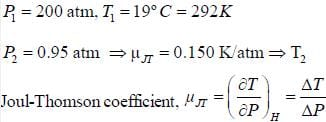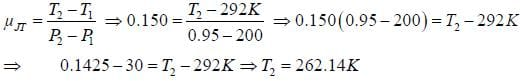Chemical Thermodynamics - Question 3

###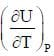is equal to

Detailed Solution for Chemical Thermodynamics - Question 3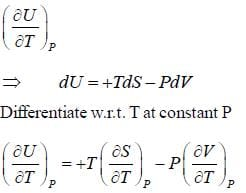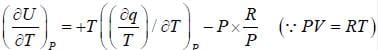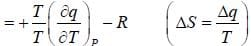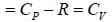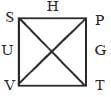*Answer can only contain numeric values
Chemical Thermodynamics - Question 4

A quantity of CH4 (g) at 298K is compressed reversibly and adiabatically from 50.0 bar to 200 bar. Assuming ideal behaviour. The value of final temperature of CH4 (g).  (Take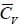= 3R ) ______________ (answer should be an integer).

Detailed Solution for Chemical Thermodynamics - Question 4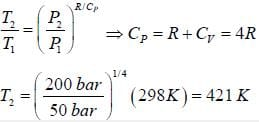Chemical Thermodynamics - Question 5

Hie change in entropy, when temperature is lower down to 1/8 of initial temperature at constant volume is

Detailed Solution for Chemical Thermodynamics - Question 5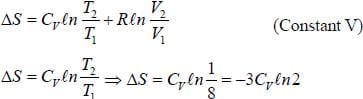*Answer can only contain numeric values
Chemical Thermodynamics - Question 6

The change in enthalpy when 5 mole of liquid benzene transforms to the vapour state at its boiling temperature(90°C) and at 1 bar pressure is (ΔSV = 87J K -mol) _____________________KJ. (Round off to two decimal places).

Detailed Solution for Chemical Thermodynamics - Question 6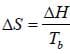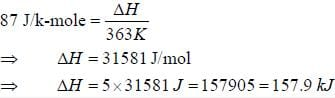Chemical Thermodynamics - Question 7

A steam engine operates between 400K and 300K under higher pressure. The minimum amount of heat that must be with drawn from the hot reservoir to obtain 1000 J of work is

Detailed Solution for Chemical Thermodynamics - Question 7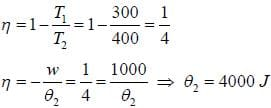Chemical Thermodynamics - Question 8

For a vander Waal gas of n mole, the expression for ΔH is

Detailed Solution for Chemical Thermodynamics - Question 8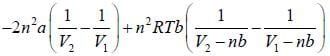Chemical Thermodynamics - Question 9

The change in entropy if one mole of S02(g) at 300K and 1.00 bar is heated to 1000K and its pressure is decreased to 0.010 bar. The molar heat InCorrect capacity of SC2(g) to be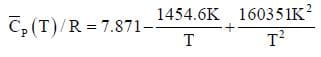Detailed Solution for Chemical Thermodynamics - Question 9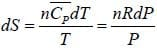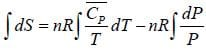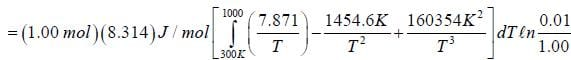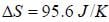Chemical Thermodynamics - Question 10

The change in entropy for the following transformation is respectively (+ indicates increases. - indicates decreases and 0 indicates no change)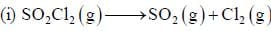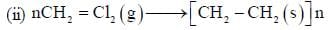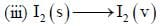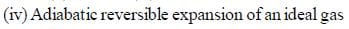Detailed Solution for Chemical Thermodynamics - Question 10

(i) Change in entropy = positive
(ii) Change in entropy = negative
(iii) Change in entropy = positive
(iv) Change in entropy = zero

Chemical Thermodynamics - Question 11

A gas obeys the equation P (Vm - b) = RT.  For this gas  b = 0.0391 dm3 mol-1 .The fugacity coefficient for the gas at 1000°C and 1000 atm is

Detailed Solution for Chemical Thermodynamics - Question 11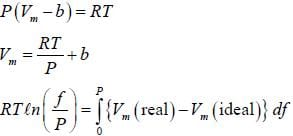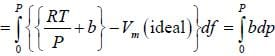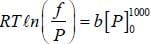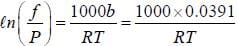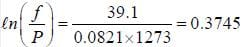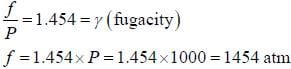Chemical Thermodynamics - Question 12

The correct relation for ΔU in van der Waal gas (in isothermal variation ) is

Detailed Solution for Chemical Thermodynamics - Question 12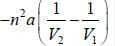Chemical Thermodynamics - Question 13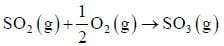is exothermic by 97030 J at 298K and 1 atm measured in a bomb calorimeter. The value of ΔH is

Detailed Solution for Chemical Thermodynamics - Question 13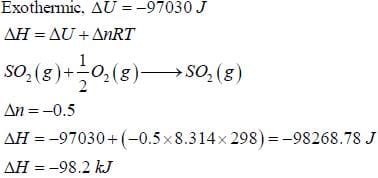*Answer can only contain numeric values
Chemical Thermodynamics - Question 14

The ΔGmix  when 10 moles of Ne get mixed with 20 moles of equimolar mixture of He and Ne is ____________kJ.(Round off to one decimal place)

Detailed Solution for Chemical Thermodynamics - Question 14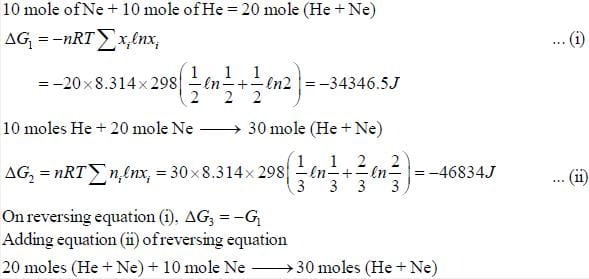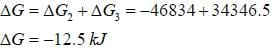*Answer can only contain numeric values
Chemical Thermodynamics - Question 15

A system filled with 0.505 mol of gas contracts reversibly from 1L to 0.10 L at constant temperature at 5°C. It simultaneously loses 1270 J of heat. The ΔU is____________J. (Round off to nearest integer))

Detailed Solution for Chemical Thermodynamics - Question 15

q = -1270J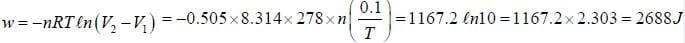Even through process is isothermal but overall ΔU change is not zero. So. applying first law
ΔU = q + w = -1270 + 2688 = 14187

*Answer can only contain numeric values
Chemical Thermodynamics - Question 16

The molar heat capacity at constant pressure of  H2O (g) at 1.00 atm is given by
Cp =30.54 + 10.3 x 10-3 T
The change in entropy when 2.0 mole of water at 1.00 atm. The temperature of H2O(g) is increased from 300K to 1000K is____________J K. (Round off to two decimal places)

Detailed Solution for Chemical Thermodynamics - Question 16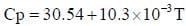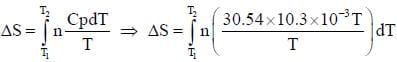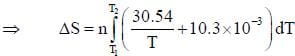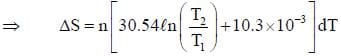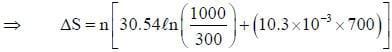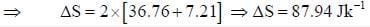Chemical Thermodynamics - Question 17

The molecular partition function of a system is given by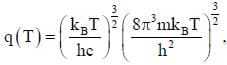where the symbols have their usual meanings. The heat capacity at constant volume for this system is

Detailed Solution for Chemical Thermodynamics - Question 17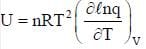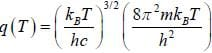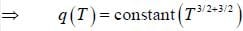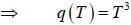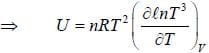Chemical Thermodynamics - Question 18

1 mole of N2, 2 mole of O2 and 1 mole of CO2 were mixed at 300K. The entropy of mixing is

Detailed Solution for Chemical Thermodynamics - Question 18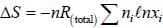'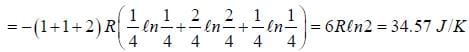Chemical Thermodynamics - Question 19

One mole of an ideal ga s Cv = 1.5 R at temperature 40 0K is com pressed from 2 atm to 3 atm by a reversible isothermal path. Subsequantly, it is expanded back to 2 atm by a reversible adiabatic path. The value of final state in litre is

Detailed Solution for Chemical Thermodynamics - Question 19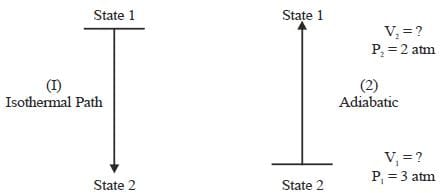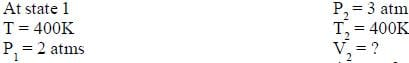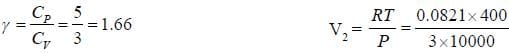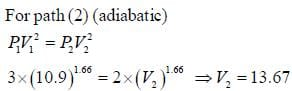Chemical Thermodynamics - Question 20

Choose the correct option in the favour of exact differential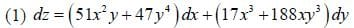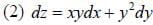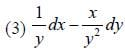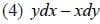Detailed Solution for Chemical Thermodynamics - Question 20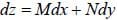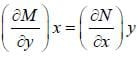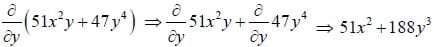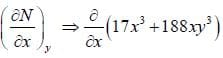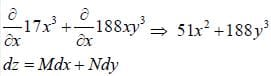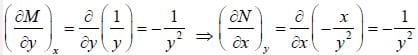## GATE Chemistry Mock Test Series

1 docs|34 tests
 Use Code STAYHOME200 and get INR 200 additional OFF Use Coupon Code